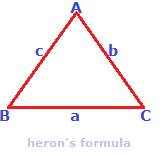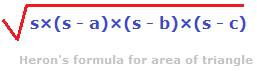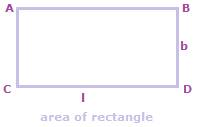## How to calculate area

How to calculate area of:

• A triangle, when the length of its three sides are given
• A square, when the length of its diagonal is given
• A rectangle, the lengths of whose diagonal and perimeter are given

Question:

How to calculate area of a triangle if the lengths of its three sides are given?Use heron’s formula to calculate area of a triangle when the lengths of its three sides are given.

And, Heron’s formula isIn the formula, a, b and c denote the lengths of the three sides of the triangle; and s is the semi-perimeter of the triangle, i.e.

s = (a+ b + c)/2

For example, the area of a triangle in which the lengths of the three sides are 6, 8 and 10 isHow to calculate area of a square when its diagonal length is given?

To calculate area of a square using its diagonal, the formula isThe area of a square whose diagonal length is 10 cms is

102/2 = 100/2 = 50 sq. cms.

How to calculate area of a rectangle whose perimeter and diagonal lengths are given?In a rectangle, if length is l and breadth is b, then

Perimeter of the rectangle = 2 (l + b) and

Diagonal length = √(l2 + b2)

Apply the algebraic identity below, to calculate area of the rectangle:

(l + b)2 = l2 + b2 + 2lb,

Transpose l2 + b2 to the other side, so

2lb = (l + b)2 – ( l2 + b2),

Next divide by 2 to finally calculate area of the rectangle as below:

l× b = ((l + b)2 – (l2 + b2))/2

Related Topics

Area of Triangle